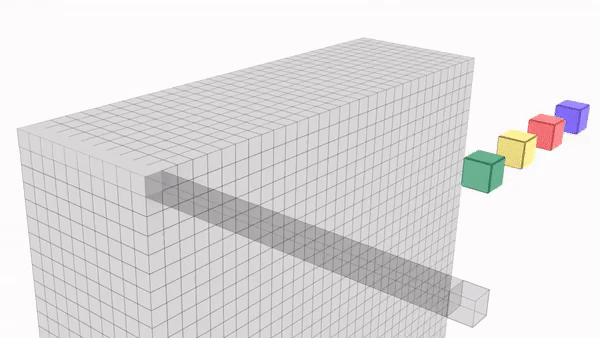# 6. Introduction to Deep Learning with Computer Vision — 3×3 is a lie! 1×1 convolutions

Source: Deep Learning on Medium# 6. Introduction to Deep Learning with Computer Vision — 3×3 is a lie! 1×1 convolutions

written by Nilesh Singh and Praveen Kumar.

Prerequisites: Filters & Kernels, Channels & Features

So far, we’ve looked at a very specific type of convolution with a kernel size of 3, more commonly called 3X3 convolutions. We also said that this the only type of convolution that we are going to use. But what if I told you that we were indeed lying.

There is a very important kind of convolution that is under some circumstances more important than our feature extractor 3X3.

Before looking at what it is, let’s look at the problem faced with 3X3 convolutions.

Let’s say that we have an image with some arbitrary size, also let’s assume that we are doing a series of feature extractions on it using 3X3 kernels. We know that it is in the best interest of the model to increase the number of channels in each convolutional layer.

So, if we generate 32 channels in 1st convolution then our GPU will have 32 images after 1st convolution. In the second iteration if we generate 64 channels, then for each of 32 images generated in the previous step 64 new channels will be generated.

total no. of images in GPU=32X64

if in the 3rd convolution, we generate 128 channels, then no of images=32X64X128.

We have networks with 1000s of such layers, just try to imagine the number of images that GPU will have to handle. Even state of art Titan X GPUs is tiny when confronted with such a huge number.

What do we do?